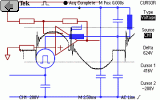Electrotechnics

# CMOS Oscillating Circuitswww.e-rokodelnica.si

Despite their venerable years, the CMOS gates are very handy. They could be used to build very simple and cheap oscillating circuits that are presented here. It is shown how to calculate frequency. For help, a java script calculator is added to each circuit, so that we can obtain period of oscillations easily.

## One Gate Oscillator

The simplest oscillating circuit could be build around an inverting buffer with Schmitt trigger input. This input is used to lower buffer's sensitivity to disturbances. Especially in case of an input signal that has a very slow slew rate. Then, we would get many switching events near the switching point of circuit which has no Schmitt trigger input.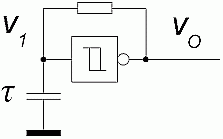Figure 1.1: Schematics

As we can see in the Figure 1.2, the buffer input oscillates between both threshold voltages VL and VH of Schmitt trigger input. Actually, this hysteresis makes oscillating possible.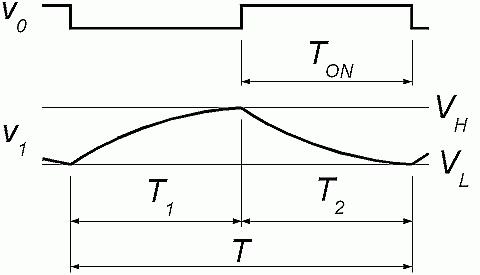Figure 1.2: Signals

To get a period T, we must figure out rise and fall times of capacitor voltage. First, we calculate rise time T1 from lower threshold VL to upper threshold VH. The time depends on threshold voltages and time constant of RC circuit. The equation is one of the basic equations in electronics.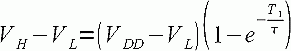(1.1)

Similarly, we obtain fall time T2 of voltage from upper threshold VH to lower threshold VL.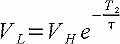(1.2)

Now, both times T1 and T2 are added to obtain a period of oscillations.

In the period calculator, normalized voltages v and times t are used. All voltages in the Equations 1.1 and 1.2 are divided by the supply voltage VDD and all the times are divided by time constant RC (tau). These definitions can be found in the Table 1 also.

The following Equations 1.3 and 1.4 are normalized version of the Equations 1.1 and 1.2.(1.3)(1.4)

To use the oscillator period calculator, the normalized threshold voltages must be obtained. The two are filled in the first two fields of the form in the Table 1. After that, we click the calculate button. The results are also normalized. Therefore, we must multiply the normalized period by time constant RC (tau) to obtain the real period of oscillations (i.e. Frequency).

 Variable Value Positive Trigger Threshold Voltage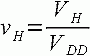Negative Trigger Threshold Voltage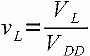Press the button to calculate the period! Intermediate result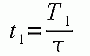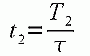Duty Cycle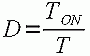Period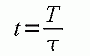The oscillator is very cheap and reliable. But, it is extremely sensitive to threshold voltages VL and VH. Different voltages give very different periods and also duty cycles. Unfortunately, these voltages could be very different among devices, especially when we got them from different vendors.

Do not use this circuit when frequency or duty cycle is important!

## Double RC Oscillator

This oscillator circuit consists of three inverter buffers and two equal RC stages. The frequency is still very sensitive to threshold voltages VL, VH and may vary a lot. But its duty cycle is much more stable.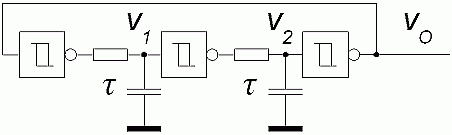Figure 2.1: Schematics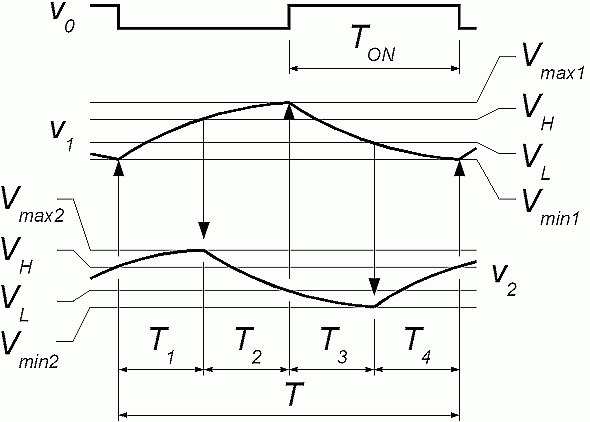Figure 2.2: Signals

As we can see from the signal diagrams, voltages at gate inputs pass beyond the threshold voltages. We got four peak voltages that are unknown at a moment. Additionally, we got four unknown time periods. To solve for time periods, we need eight Equations form 2.1 to 2.8. These Equations are equations of charging and discharging of capacitors.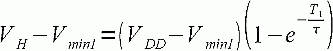(2.1)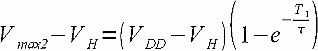(2.2)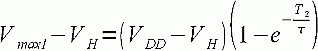(2.3)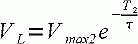(2.4)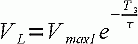(2.5)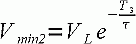(2.6)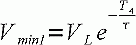(2.7)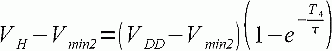(2.8)

The equations are rearranged to cancel unknown voltages out. Here are four Equations from 2.9 to 2.12 left.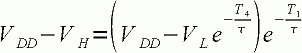(2.9)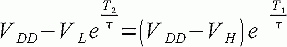(2.10)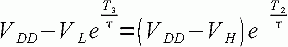(2.11)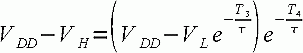(2.12)

In the period calculator, normalized voltages v and times t are used. All voltages in the Equations 2.9 and 2.12 are divided by the supply voltage VDD and all the time periods are divided by time constant RC (tau). These definitions can be found in the Table 2 also.

The following Equations 2.13 and 2.16 are normalized version of the Equations 2.9 and 2.12.(2.13)(2.14)(2.15)(2.16)

Now, some tedious work is to be done to turn these equations around to get time periods. The solution is hidden inside of the oscillator period calculator in the Table 2. To use the calculator, the normalized threshold voltages must be obtained. The two are filled in the first two fields of the form in the Table 2. After that, we click the calculate button. The results are also normalized. Therefore, we must multiply the normalized period by time constant RC (tau) to obtain the real period of oscillations (i.e. Frequency).

 Variable Value Positive Trigger Threshold VoltageNegative Trigger Threshold VoltagePress the button to calculate the period! Intermediate result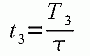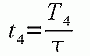Duty CyclePeriodThis circuit may be used in case when a stable 50% duty cycle is important. But on the other hand, frequency may vary.